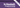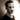Folding ListsA whole chapter dedicated to folding lists? I thought it would be quick and easy, but it turns out there's a lot of things to uncover in regards to folding. In this post we'll be looking at what a fold is and how folding left differs from folding right.

## A brief explanation of folding

Before we can talk about folding left or right, it's important to know what we're talking about. In short, a fold is a way to reduce a data structure (lists in our case) to some other type by working through the data structure. If that sounds unclear, a more concrete example could be a function that sums all the numbers in a list or a function which tells you whether any element of a list satisfies a predicate.

Let's look at `foldr`, short for 'fold right'. We'll talk about `foldl` (fold left) in a bit. The signature for `foldr` is as follows as taken from GHCi, version 8.6.5:

``````class Foldable (t :: * -> *) where
foldr :: (a -> b -> b) -> b -> t a -> b``````

We haven't talked about kinds yet (that's in the next chapter), but the `t :: * -> *` part means that `t` is a type that is parameterized by another type. In our instance, we can substitute it with a list instead:

``foldr :: (a -> b -> b) -> b -> [a] -> b``

So `foldr` is a higher-order function that relies on two parametrically polymorphic types, `a` and `b`. It takes a function from `a` and `b` to `b`, an initial `b` value, a list (or `Foldable`) of `a`, and returns a `b`.

This snippet is taken from the Haskell Wiki article on folding (which I recommend you take a look at if you're not quite familiar with it), and shows the equations defining `foldr`:

``````-- if the list is empty, the result is the initial value z; else
-- apply f to the first element and the result of folding the rest
foldr f z []     = z
foldr f z (x:xs) = f x (foldr f z xs)``````

In the above snippet `f` is the `(a -> b -> b)`, `z` is the `b`, and the `[a]` is either destructured into `[]` or `(x:xs)`.

Folds are catamorphisms, a way of deconstructing data, reducing a structure to a completely different result.

## Why direction matters: `foldr` vs `foldl`

Building on the basic definition of a fold, let's explore the differences between folding left and folding right and what impacts that has on your programs.

Let's revisit the definition of `foldr`, but this time put `foldl` just below it. Again, the snippet is taken from the Haskell Wiki article on folding, but I've added the function types for clarity.

``````-- if the list is empty, the result is the initial value z; else
-- apply f to the first element and the result of folding the rest
foldr :: (a -> b -> b) -> b -> [a] -> b
foldr f z []     = z
foldr f z (x:xs) = f x (foldr f z xs)

-- if the list is empty, the result is the initial value; else
-- we recurse immediately, making the new initial value the result
-- of combining the old initial value with the first element.
foldl :: (b -> a -> b) -> b -> [a] -> b
foldl f z []     = z
foldl f z (x:xs) = foldl f (f z x) xs``````

These two functions are nearly identical, but they flip the order of the parameters of the first function and they behave quite differently. Notice how `foldr` applies `f` to `x` and then to the result of folding the rest of the list, while `foldl` immediately recurses before applying `f` to `z` and `x`. This may seem unimportant, but it has big ramifications in a lazy language like Haskell.

With `foldr`, if you have a function that lazily evaluates its arguments, you could potentially short-circuit the recursion long before reaching the end of the list, but with `foldl`, because the function recurses immediately, you force the evaluation of the entire list. This means that if you have an infinite list, `foldl` will never finish, while `foldr` might.

Let's see an example. If we want to use `foldr` to find whether any number in an infinite list is even, we could do it like this:

``````foldr (\x b -> even x || b) False [ 1 .. ]
-- returns True``````

Even if the list is infinite, the function we pass to `foldr` doesn't evaluate its second parameter (`b`) if `even x` evaluates to `True`. In the example above, the evaluation would work a little something like this:

``````-- the list is not empty, so apply f to the head of the list
-- and set up potential recursion
even 1 || foldr (\x b -> even x || b) False [ 2 .. ]
-- even 1 is False, so we take another step and recurse.

even 2 || foldr (\x b -> even x || b) False [ 3 .. ]
-- even 2 is True, so we don't need to evaluate anymore.
-- we can happily return the result with only two steps.``````

In this example, the function can exit after only two steps through the list because our function (`\x b -> even x || b`) doesn't evaluate `b` unless `even x` is False. If we look at the same example with `foldl`, however, the result is quite different:

``````-- note the flipped parameter order for f
foldl (\b x -> even x || b) False [ 1 .. ]
-- ... ... ... ...
-- never terminates``````

Because `foldl` recurses unconditionally (`foldl f (f z x) xs`), the function will start to evaluate, but because it needs to get to the end of the list before it can evaluate the function and because the list is infinite, the function can never terminate.

`foldr`, on the other hand, doesn't recurse immediately, and flips back and forth between evaluating `f` and taking another step. This means that, because of lazy evaluation, `foldr` can stop recursing through the list after any number iterations, depending on the function.

Let's relate back to what we talked about last time and talk about spines. Folding happens in two stages: traversal and folding. Traversal is the stage in which the fold recurses over the spine. Folding is the evaluation of the folding function applied to the values. All folds recurse over the spine in the same direction. However, the difference lies in the association of the folding function and direction the folding proceeds. This sounds tricky, but we can demonstrate it by parenthesizing:

``````foldr (+) 0 [ 1 .. 5 ]
-- evaluates as the following
-- (1+(2+(3+(4+(5+0)))))

foldl (+) 0 [ 1 .. 5 ]
-- evaluates as the following
-- (((((0+1)+2)+3)+4)+5)``````

Notice how the order in which the expressions are evaluated is reversed, as is the end at which the zero element is applied. If we use a non-commutative operation instead of summing, we'll see the last point more clearly:

``````foldr (++) "0" ["a", "b", "c"]
-- evaluates to "abc0"

foldl (++) "0" ["a", "b", "c"]
-- evaluates to "0abc"``````

Notice how the `0` ends up at opposite ends of the result because of the evaluation order.

### Folding summary

In short, the book recommends to always use `foldr` over `foldl` and provides a helpful summary:

• `foldr` ::
1. The recursive invocation of `foldr` is an argument to the folding function. It doesn't directly self-call as a tail call the way `foldl` does. In a way, you can think of `foldr` as alternating between applications of the folding function `f` and `foldr`, where the next invocation of `foldr` is dependent on `f` asking for it.
2. Is right-associative.
3. Works with infinite lists.
4. Is a good default choice for transforming data structures.
• `foldl` ::
1. Self-calls (tail call) through the list, starts to produce values after reaching the very end.
2. Is left-associative.
3. Cannot be used with infinite lists.
4. Is 'nearly useless' and should almost always be replaced with `foldl'~. We haven't talked about ~foldl'~, but it works the same as ~foldl` except it is strict, meaning it evaluates the values inside the cons-cells as it traverses the list, rather than leaving them as unevaluated expressions. We'll talk more about this when we get to the chapter on efficient code.

## Definitions

Fold
A higher-order function that takes an accumulating function and a recursive data structure and returns the value that results from applying the function to the elements in the structure.
Catamorphism
A generalization of folds to arbitrary data types. A morphism is a transformation, and cata means 'down' or 'against', so a catamorphism is a transformation to a 'lower' form.
Tail call
A tail call is the final result of a function, i.e. the last function that gets called in a function. In the below example---assuming `g` and `h` are functions---~h~ is the tail call.
``f x y = h (g x y)``
Tail recursion
a function whose tail calls are recursive invocations of itself. In our definitions of `foldr` and `foldl`, we see that `foldl` is tail recursive, while `foldr` is not, as the latter alternates between calling `f` and `foldr`.

## Summary

In addition to going deep on folding, this chapter also touches briefly on the scan functions `scanl` and `scanr`. In the interest of time I won't cover them here (unless we need them later), but if you're interested you can go check out Hackage for more information.

Next chapter is on Algebraic Data Types, and it's a big one, so stay tuned.Thomas Heartman is a developer, writer, speaker, and one of those odd people who enjoy lifting heavy things and putting them back down again. Preferably with others. Doing his best to gain and share as much knowledge as possible.